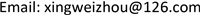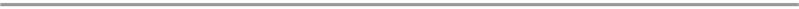﻿ 基于旱情预报条件下的应急调水研究 Emergency Water Diversion Based on Drought Forecasting

Journal of Water Resources Research
Vol.06 No.06(2017), Article ID:22395,9 pages
10.12677/JWRR.2017.66068

Emergency Water Diversion Based on Drought Forecasting

Weizhou Xing

Handan Hydrology and Water Resources Survey Bureau, Handan HebeiReceived: Oct. 3rd, 2017; accepted: Oct. 12th, 2017; published: Oct. 20th, 2017ABSTRACT

For severe water shortage areas, it is necessary for regional water transfer to be restricted by underground water pressure during the severe drought season. In view of the low water resources, the optional water source limitation and the relative increase of water transfer cost, the water transfer time must be accurately controlled in order to maximize the limited water resources. In agricultural irrigation, a mathematical model is established based on the soil moisture monitoring data, crop growth, dry time prediction of crop, and the emergency water diversion water canal characteristics. The point implementation of precision irrigation is used to minimize water loss and maximize water diversion irrigation efficiency.

Keywords:Soil Moisture Monitoring, Soil Moisture Content, Irrigation, Water Transfer Model1. 引言

2. 土壤墒情监测与预报

2.1. 墒情监测站网

2.2. 墒情监测要素Table 1. Statistical table of soil moisture monitoring station network in Handan city

(1) 气象要素。降水量、气温、气压、湿度、风速、水面蒸发量、地温、日照为主要气象观测要素。

(2) 作物生长发育状况及墒情要素。收集国家和地方的墒情监测站点代表区域的作物种植情况，即作物的分布情况、作物的种类及各种作物的所占比重。收集不同作物、不同生长期的适宜土壤含水量资料，其数值一般以土壤相对湿度来表示。收集不同作物不同生长期脱墒(缺水)和受旱的临界含水量资料，记录脱墒和受旱开始的日期、受旱的天数、代表区域干旱程度及干旱的分布情况。记录作物的播种日期、作物生长发育期，观察作物的生长发育状况，同时观测土壤含水量。

(3) 土壤的物理特性及土壤含水量。土壤的物理特性由土壤的质地、土壤的结构、土壤的比重、土壤干容重、土壤孔隙度来表达。土壤水分常数是土壤水分特性的重要指标，主要有饱和含水量、田间持水量、凋萎含水量及作物不同生长期适宜的含水量。土壤含水量是墒情和旱情监测的主要指标，土壤水分状态可由重量含水量、体积含水量、土层中的蓄水量和土壤相对湿度四个指标来表达。

2.3. 墒情预报模型

$R=P-I$ (1)

$I={I}_{m}-{P}_{a}$ (2)

${P}_{a,t+1}=K×{P}_{a,t}$ (3)

${P}_{a,t+1}=K×\left({P}_{a,t}+{P}_{t}\right)$ (4)

${\theta }_{a,t+1}={K}_{t}\left({\theta }_{a,t}+{P}_{t}+{q}_{t}\right)$ (5)

2.4. 土壤墒情预报方法

(1) 土壤含水量计算 

${W}_{b}=\frac{A-W}{W-{W}_{环}}×100%$ (6)Table 2. Calculated soil regression coefficients at Heilonggang plainTable 3. Calculated soil regression coefficients at Piedmont plain

${W}_{m}=\frac{B-W}{W-{W}_{环}}×100%$ (7)

${W}_{t}=\frac{C-W}{W-{W}_{环}}×100%$ (8)

(2) 不同土壤类型的水分常数

(3) 主要农作物适宜土壤含水量Table 5. Soil water content suitable for main crops

(4) 土壤含水量预报模型

${\theta }_{a,t+n}={K}_{t}^{n}\left({\theta }_{a,t}+{P}_{t}+{Q}_{t}\right)$ (9)

${\theta }_{a,t+5}={K}_{1}^{5}×{\theta }_{a,t}$ (10)

${\theta }_{a,t+8}={K}_{2}^{3}×\left({\theta }_{a,t+5}+{P}_{t+5}\right)$ (11)

(5) 灌水时间预测模型

$n=\frac{\mathrm{lg}\left({\theta }_{a,t+n}\right)-\mathrm{lg}\left({\theta }_{a,t}+{P}_{t}\right)}{\mathrm{lg}{K}_{t}}$ (12)

${P}_{t}=\frac{P}{h}×100%$ (13)

$n=\left(\mathrm{lg}0.21-\mathrm{lg}0.226\right)/\mathrm{lg}0.991=8\left(天\right)$ (14)

3. 输水时间计算

$T=\frac{L}{v}$ (15)

$v=C\sqrt{RJ}$ (16)

$R=\frac{A}{{P}_{w}}$ (17)

$T=k\eta L/\left({R}^{2/3}{J}^{1/2}\right)$ (18)

4. 干旱时期水网应急调水模型研究

4.1. 应急调水模型的建立

$\left\{\begin{array}{l}n=\frac{\mathrm{lg}\left({\theta }_{a,t+n}\right)-\mathrm{lg}\left({\theta }_{a,t}+{P}_{t}\right)}{\mathrm{lg}{K}_{t}}\\ T=k\eta L/\left({R}^{2/3}{J}^{1/2}\right)\\ n\le T\end{array}$ (19)

4.2. 模型的应用

(1) 实用条件：该模型应用于干旱时期，是特殊气候环境条件下为保证农业灌溉用水的应急措施。用于平原区小区域范围调水，输水河渠通畅、无污染，一般输水时间在3~10天左右，季节特征表现为气温较高，土壤含水量变化较大，少雨。根据旱情发生地与供水水源的距离推算出输水时间，结合旱情的监测数据，以输水时间

T为依据推算最低土壤含水量 ${\theta }_{a,t+n}$ 的临界值，以保证在无降雨时作物生长需要的最低土壤含水量 ${\theta }_{a,t+n}$ ，以减少旱情对农作物的损失最小。

(2) 应用举例：2009年4月某日曲周县城关陈庄村有旱情，目前土壤体积含水量为23.3%，作物处于生长期，要求土壤含水量不低于21%，需从岳城水库调水，为保证农作物不受影响，计算曲周县城关陈庄村土壤含水量达到多少时，岳城水库开始提闸放水？

$\left\{\begin{array}{l}n=\frac{\mathrm{lg}\left({\theta }_{a,t+n}\right)-\mathrm{lg}\left({\theta }_{a,t}+{P}_{t}\right)}{\mathrm{lg}{K}_{t}}\\ T=k\eta L/\left({R}^{2/3}{J}^{1/2}\right)\\ n\le T\end{array}$

5. 结论

Emergency Water Diversion Based on Drought Forecasting[J]. 水资源研究, 2017, 06(06): 585-593. http://dx.doi.org/10.12677/JWRR.2017.66068

1. 1. 中华人民共和国行业标准. (SL000-2005)土壤墒情监测规范[S]. 北京: 中国水利水电出版社, 2005. People’s Republic of China Industry Standard. (SL000-2005) Soil moisture monitoring standard. Beijing: China Water Conservancy and Hydropower Press, 2005.

2. 2. 乔光建. 北方干旱地区土壤墒情预报模型[J]. 南水北调与水利科技, 2009, 7(1): 39-42. QIAO Guangjian. Prediction model of soil moisture content in arid area of North China. South to North Water Transfer and Water Conservancy Science and Technology, 2009, 7(1): 39-42.

3. 3. 关连珠. 普通土壤学[M]. 北京: 中国农业大学出版社, 2007: 37-57. GUAN Lianzhu. General soil science. Beijing: China Agricultural University Press, 2007: 37-57.

4. 4. 王振龙, 高建峰. 实用土壤商情检测预报技术[M]. 北京: 中国水利水电出版社, 2006. WANG Zhenlong, GAO Jianfeng. Soil in practical detection and prediction technology. Beijing: China Water Conservancy and Hydropower Press, 2006.